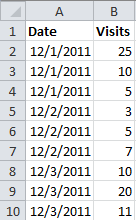# VBA

## Applying Excel Subtotals with VBA

Excel’s subtotal option (Data –> Subtotal) lets you display a subtotal on any number of columns for each change in a particular column. This is useful for sheets where each day, for instance, might have multiple entries recording some bit of data, and you want subtotals for each day giving you the sum, or the min, or max, or some other aggregate. Say you have something like this visit-tracking data, sorted by Date:If you choose Data –> Subtotal, you get this dialog, which you use to define which column Excel should check for changes, and which column(s) should get a subtotal, and what sort of subtotal it should be (e.g. SUM, COUNT, AVERAGE, MAX, MIN, etc.):After pressing the OK button and adjusting column widths, your sheet looks like this:You can see that Excel adds subtotal lines for each change in date, as well as a grand total line at the bottom. If you single-click B5, B9, B13, and B14, you’ll see that Excel has written SUBTOTAL() functions for each of the three ranges created by the changes in the Date column, and has applied the same function to the entire range to get the grand total.

You can turn them back off by opening the Subtotal dialog a second time and clicking the Remove All button instead.

That’s handy, but wouldn’t it be handier to turn this on and off via VBA? I thought so, and it’s pretty easy to do. Fire up the VBA Editor (Developer –> Code –> Visual Basic), double-click the sheet which contains your data (e.g. Sheet1) from the Project Explorer on the left, and create two subroutines. The first, AddSubs() will turn on the subtotals. A second, RemoveSubs() will turn turn them off. Here is the code for AddSubs():

```Sub AddSubs()
Worksheets("Sheet1").Activate
Selection.Subtotal GroupBy:=1, Function:=xlSum, TotalList:=Array(2)
End Sub```

It’s pretty simple. You can see that the worksheet is specified by name in the second line. In the third line, the GroupBy value (i.e. 1), is used to specify the column where Excel should look for changes. In VBA, the columns are numbered from left to right. So 1 is Column A, our Date column. The TotalList array specifies the columns which should get a subtotal. So 2 is Column B, our Visits column. If you have more than one of them, just separate each number with a comma (e.g. Array(2, 3)). The Function parameter tells Excel what sort of subtotal to create. You feed it one of several contants (e.g. xlSum, xlMin, xlMax, etc.).

Turning subtotals off is equally easy. So long as you get the worksheet name right, you should be in business. Here’s the code for RemoveSubs():

```Sub RemoveSubs()
Worksheets("Sheet1").Activate
Selection.RemoveSubtotal
End Sub ```

To use either one, click your mouse inside any of the cells that contain your data (here, any cell in the A1:B10 range) choose Developer –> Code –> Macros, select the macro from the list, and press the Run button.